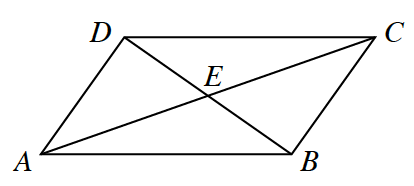### Home > CCGS > Chapter 11 > Lesson 11.1.3 > Problem11-48

11-48.En el diagrama de la derecha, asume que $m∠ECB=m∠EAD$ y el punto $E$ es el punto medio de $\overline{AC}$. Demuestra que $\overline{AD}\cong\overline{CB}$.

Si puedes probar que el $\Delta DEA \cong \Delta CEB$, entonces, puedes probar que $\overline{AD}\cong\overline{CB}$.

Puedes probar que el $\Delta DEA \cong \Delta CEB$ por $≅\text{AAL}$.

Flowchart, 6 bubbles, Bubbles 1, 2, 3 on top row, 4, & 5 on middle row, and 6, on bottom row, with arrows as follows: from 1 to 4. From 2 to 4, from 3 to 5, from 5 to 4, from 4 to 6.

 Burbuja 1$\enclose{circle}{ \; \\ \quad m \angle ECB = m \angle EAD \quad \\}$ Burbuja 2$\enclose{circle}{ \; \\ \quad m \angle AED = m \angle CEB \quad \\}$ Burbuja 3$\enclose{circle}{ \; \\ \; \\ \quad E \; \text{is the midpoint} \quad \\ \qquad \quad \text{of} \; \overline{AC}\\}$ $\text{Dado} \; \Huge \searrow$ ${\text{Vertical} \; \\ \text{angles are} \; \\ \text{congruent}}\; \Huge \downarrow$ ${\Huge \downarrow} \; \text{Dado}$ Burbuja 4$\enclose{circle}{ \; \\ \quad \Delta AED \; \cong \; \Delta CEB \quad \\}$ $\Huge \leftarrow$ Burbuja 5$\begin{array}{c} \enclose{circle}{ \; \\ \quad \overline{AE} \; \cong \; \overline{CE} \quad \\} \\ \text{Def. of midpoint} \end{array}$ ${\Huge \downarrow} \; \text{ASA}\cong$ Burbuja 6$\begin{array}{l} \enclose{circle}{ \; \\ \quad \overline{AD} \; \cong \; \overline{CB} \quad \\} \\ \qquad \qquad \quad \Delta \text{s} \cong \; \rightarrow \text{partes} \cong \end{array}$